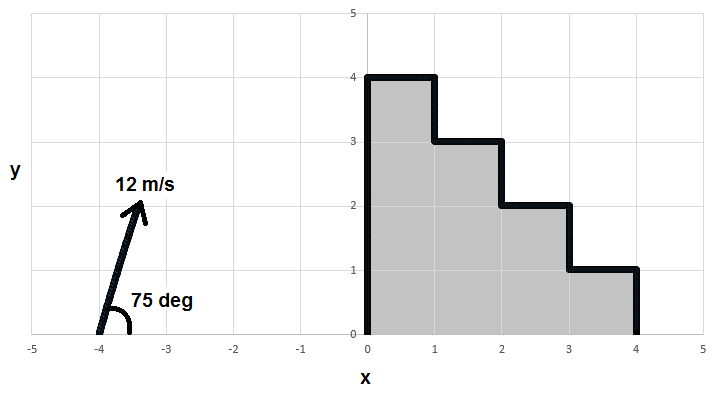# Which Step Does It Land On?A projectile is launched from an initial position $(x,y) = (-\SI{4}{\meter},\SI{0}{\meter})$. The projectile's initial speed is $12\text{ m/s}$ and it is launched at a $\SI{75}{\degree}$ angle with respect to the $x$-axis. There is a staircase with 4 steps, as pictured above. The top step begins at $(x,y) = (\SI{0}{\meter},\SI{4}{\meter})$ meters and the bottom step ends at $(x,y) = (\SI{4}{\meter},\SI{0}{\meter})$. Each step is $\SI{1}{\meter}$ wide and $\SI{1}{\meter}$ deep. The projectile flies over part of the staircase and lands on one of the steps. What is the $x$ coordinate of the landing point in meters?

Details and Assumptions

• The gravitational acceleration is in the $-y$ direction, with a magnitude of $\SI{9.8}{\meter\per\second\squared}$.
×